# Integral cosine

The special function defined, for real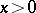, by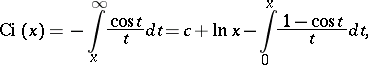where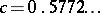is the Euler constant. Its graph is: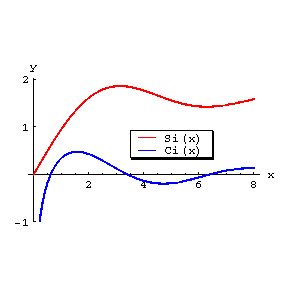Figure: i051370a

The graphs of the functionsand.

Some integrals related to the integral cosine are: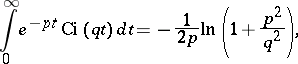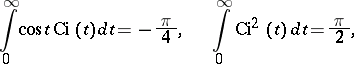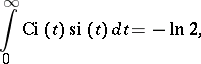whereis the integral sine minus.

Forsmall: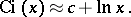The asymptotic representation, forlarge, is: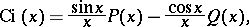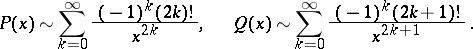The integral cosine has the series representation: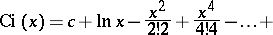(*)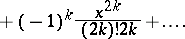As a function of the complex variable,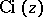, defined by (*), is a single-valued analytic function in the-plane with slit along the relative negative real axis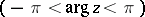. The value of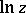here is taken to be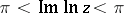. The behaviour ofnear the slit is determined by the limits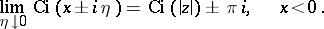The integral cosine is related to the integral exponential function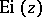byOne sometimes uses the notation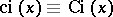.